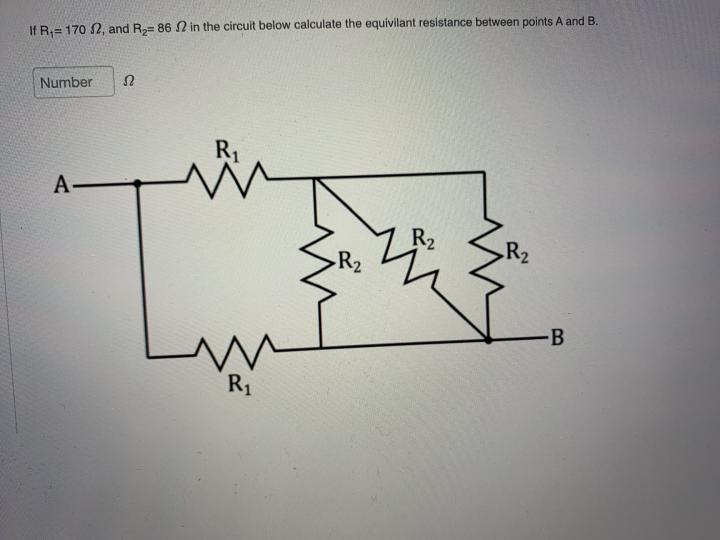# Question IR,= 1702, and R = 86 in the circuit below calculate the equivilant resistance between points A and B. Number Ω Ri A M R2 -R2 SRL R2 M Kan R1